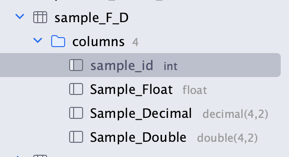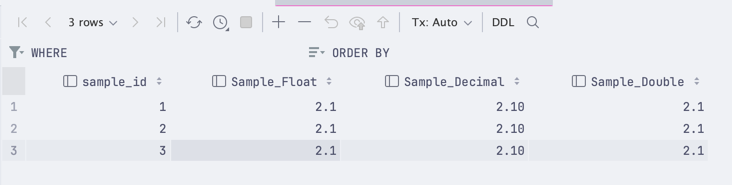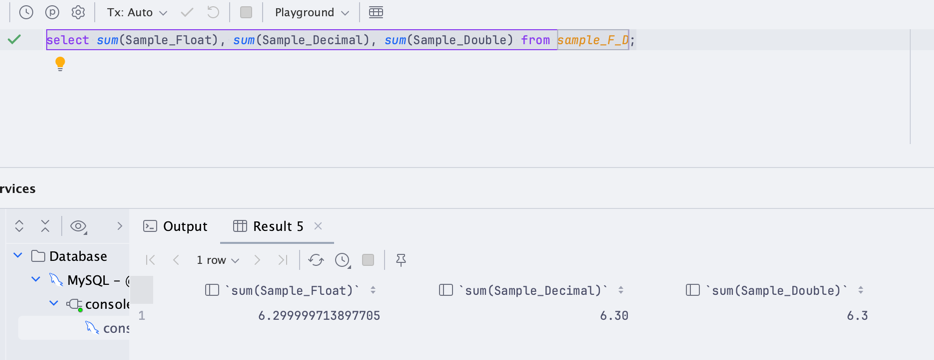# MySQL: Decimal vs Double vs Float?

When deciding how to store your data in MySQL - you might see a few options. Especially if you look at float variations:

• `float`
• `double`
• `decimal`

But which one should you use? What's the difference? Let's take a look at these fields and see where they can be used:

Our example database:And here's the data we have stored in it:Now, let's try to sum the total on each of these fields:As you can see, it quickly shows us that `float` is the least precise, while `decimal` is the most precise. So, what's the use case of this?

• `float` and `double` are good for calculations where you don't have to be really precise. These values will not always come up to an exact precision, but they are fast to calculate.
• `decimal` is suitable for calculations where you need to be precise. These values will always come up to an exact precision, but they are slower to calculate.

That said, here's the typical use case for each of these fields that we have seen:

• Geo Coordinates - `double`, as you don't need to be really precise, but you need to be fast and store a lot of decimal places
• Money - `decimal`, as you need to be precise, but you don't need to be fast. You can, and should store money in cents, but this is a backup idea
• Statistics - `float/double` or `decimal`, as this depends on how precise you need to be. If you are okay being off by a few decimal places - use `float`, if you need to be precise - use `decimal`.
• Stock values (especially if products can be sold in measurements) - `decimal`, as you need to be precise.
• Distance - `double` if you don't need to be precise, `decimal` if you need to be precise. For example, you might be okay with being off by a few meters using `double`.
• Measurements - `decimal`, as you need to be precise.
• Percentages - `decimal`, as you need to be precise. Especially if it is sensitive data, like money.

Of course, all of these depend on your case, and there is no one-size-fits-all solution. But keep in mind that Float has quite a few issues with it and shares them with Double. So, if you need to be more precise - use Decimal (even though it might not eliminate everything, it's better).thanks for the good tip.

👍 1Great tip about number data types.what about int? I store money in cents in int with a custom getter and setter that multiplies and devides by 100. But I am always not sure what i need to do, when I calculate taxes for example. Calculate in cents or in the getter value devided by 100By the standard - you should do all of your calculations with an `int` as that's what will give you the most correct result.

Calculating after a getter value practically removes the reason to have the cents in your database as it will still cause problems with floating points (0.01 + 0.02 = 0.03405 (random numbers))

## Like our articles?

What else you will get:
• 50 courses (910 lessons, total 46 h 42 min)
• 69 long-form tutorials (one new every week)